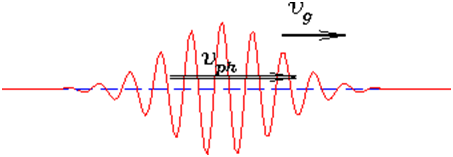# The de Broglie Equation and the Wave Nature of Matter

The de Broglie equation revealed to the world one of the most counter-intuitive concepts in Physics: wave nature of matter. You have seen examples of both particle nature of light and wave nature of light. You know about the Photoelectric effect  Albert Einstein’s courtesy. In the photoelectric effect the electrons and photons exhibit the properties of a particle, just like a billiard ball. But you surely remember the Diffraction experiment and the Interference Rings. Just like how two ripples on the surface of a pond interact. We see the wave nature of light in these cases. It’s an amazing mystery.

It even involves our sight! The gathering and focusing mechanism of light by the eye-lens conforms the wave nature of light.But its absorption by the rods and cones of the retina conforms to the particle nature of light! While we were still struggling to understand this mystery, along came Louis de Broglie to make it even more complicated with his de Broglie Relation. De Broglie’s hypothesis stated that there is  symmetry in nature and that if light and radiation behave as both particles and waves, matter too will have both a particle and wave nature.

The non-particle behaviour of the matter was proposed by a French physicist, Louis de Broglie in 1923. He was the one who discovered that particles also exhibit wave properties and derived an equation by using Planck equation and relation between mass and energy.

In fact, radiation exhibit dual nature as a wave and a particle. Hence, the wave can be expressed as:

Wavelength = h/mv

Where,

V indicates velocity

M shows mass of the particle

H is the Planck’s constant

The wavelength of the corresponding wave is known as de-Broglie wavelength.

Derivation of de-Broglie’s wavelength:

According to Planck’s quantum theory, the energy of a photon is described as

E=hυ……….(i)

Where υ denotes frequency

According to Einstein’s mass energy relation,

E=mc2……….(ii)

From both the equation, we get

hυ=mc2

hcλ=mc2

hλ=mc……….(iii)

The above equation shows the wavelength based on velocity and mass of light. When particle moves with a velocity v having a mass m is described as de-Broglie wavelength and ranges between 0 and infinity.

Hence the de-Broglie wavelength ranges between 0 and infinity.

The de-Broglie wavelength deals with the uncertainty of particles as shown in the below diagram.Moreover, de-Broglie waves are not electromagnetic because the particles have to be charged for the formation of electromagnetic waves.

The wave nature can be observed in microscopic objects. While in bigger objects, the wavelength is still smaller and becomes so small that it turns next to unnoticeable.

The wave-particle duality was one of the biggest mysteries for scientists. The light forms energy that traverses via space similar to ripples across the surface of a pond when disturbed by dropping a stone and proves wave nature of light.

## The de Broglie Equation

Λ=hp=hmv

Through the de Broglie’s relationship, we now had a wave theory of matter. The ‘Lambda’ here represents the wavelength of the particle and ‘p’ represents the momentum of the particle. The significance of de Broglie relationship is that it proves mathematically that matter can behave as a wave. In layman terms, de Broglie equation says that every moving particle – microscopic or macroscopic –has its own wavelength.

For microscopic objects, the wave nature of matter is observable. For larger objects, the wavelength gets smaller with the increasing size of the object, quickly becoming so small as to become unnoticeable which is why macroscopic objects in real life don’t show wave like properties. Even the cricket ball you throw has a wavelength which is too small for you to observe. The wavelength and the momentum in the equation are connected by the Plank’s constant.

### Heisenberg’s Uncertainty Principle

The Davisson-Germer experiment proved beyond doubt the wave nature of matter by diffracting electrons through a crystal. In 1929, de Broglie was awarded the Noble prize for his matter wave theory and for opening up a whole new field of Quantum mechanics Physics. The matter wave theory was gracefully incorporated by the Heisenberg’s Uncertainty Principle. The Uncertainty Principle states that for an electron or any other particle, both the momentum and position cannot be known accurately at the same time. There is always some uncertainty with either the position ‘σx’ or with the momentum, ‘σp’. The Heisenberg’s Uncertainty equation is.

σxsigmap≥h2

Say you measure the momentum of the particle accurately so that ‘σp’ is zero. To satisfy the equation above, the uncertainty in the position of the particle, ‘σx’ has to be infinite. From the de Broglie equation, we know that a particle with a definite momentum has a definite wavelength ‘Lambda’. A definite wavelength extends all over space all the way to infinity. By Born’s Probability Interpretation this means that the particle is not localized in space and therefore the uncertainty of position becomes infinite. In real life though, the wavelengths have a finite boundary and are not infinite and thus both the position and momentum uncertainties have a finite value. The de Broglie equation and Heisenberg’s Uncertainty Principle are apples of the same tree.